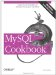# Sorting Dotted-Quad IP Values in Numeric Order

6.16.1 Problem

You want to sort strings that represent IP numbers in numeric order.

6.16.2 Solution

Break apart the strings and sort the pieces numerically. Or just use INET_ATON( ).

6.16.3 Discussion

If a table contains IP numbers represented as strings in dotted-quad notation (for example, 111.122.133.144), they'll sort lexically rather than numerically. To produce a numeric ordering instead, you can sort them as four-part values with each part sorted numerically. To accomplish this, use a technique similar to that for sorting hostnames, but with the following differences:

• Dotted quads always have four segments, so there's no need to prepend dots to the value before extracting substrings.
• Dotted quads sort left to right, so the order in which substrings are used in the ORDER BY clause is opposite to that used for hostname sorting.
• The segments of dotted-quad values are numbers, so add zero to each substring to tell MySQL to using a numeric sort rather than a lexical one.

Suppose you have a hostip table with a string-valued ip column containing IP numbers:

```mysql> SELECT ip FROM hostip ORDER BY ip;
+-----------------+
| ip |
+-----------------+
| 127.0.0.1 |
| 192.168.0.10 |
| 192.168.0.2 |
| 192.168.1.10 |
| 192.168.1.2 |
| 21.0.0.1 |
| 255.255.255.255 |
+-----------------+```

The preceding query produces output sorted in lexical order. To sort the ip values numerically, you can extract each segment and add zero to convert it to a number using an ORDER BY clause like this:

```mysql> SELECT ip FROM hostip
-> ORDER BY
-> SUBSTRING_INDEX(ip,'.',1)+0,
-> SUBSTRING_INDEX(SUBSTRING_INDEX(ip,'.',-3),'.',1)+0,
-> SUBSTRING_INDEX(SUBSTRING_INDEX(ip,'.',-2),'.',1)+0,
-> SUBSTRING_INDEX(ip,'.',-1)+0;
+-----------------+
| ip |
+-----------------+
| 21.0.0.1 |
| 127.0.0.1 |
| 192.168.0.2 |
| 192.168.0.10 |
| 192.168.1.2 |
| 192.168.1.10 |
| 255.255.255.255 |
+-----------------+```

A simpler solution is possible if you have MySQL 3.23.15 or higher. Then you can sort the IP values using the INET_ATON( ) function, which converts a network address directly to its underlying numeric form:

```mysql> SELECT ip FROM hostip ORDER BY INET_ATON(ip);
+-----------------+
| ip |
+-----------------+
| 21.0.0.1 |
| 127.0.0.1 |
| 192.168.0.2 |
| 192.168.0.10 |
| 192.168.1.2 |
| 192.168.1.10 |
| 255.255.255.255 |
+-----------------+```

If you're tempted to sort by simply adding zero to the ip value and using ORDER BY on the result, consider the values that kind of string-to-number conversion actually will produce:

```mysql> SELECT ip, ip+0 FROM hostip;
+-----------------+---------+
| ip | ip+0 |
+-----------------+---------+
| 127.0.0.1 | 127 |
| 192.168.0.2 | 192.168 |
| 192.168.0.10 | 192.168 |
| 192.168.1.2 | 192.168 |
| 192.168.1.10 | 192.168 |
| 255.255.255.255 | 255.255 |
| 21.0.0.1 | 21 |
+-----------------+---------+```

The conversion retains only as much of each value as can be interpreted as a valid number. The remainder would be unavailable for sorting purposes, each though it's necessary to produce a correct ordering.MySQL Cookbook
ISBN: 059652708X
EAN: 2147483647
Year: 2005
Pages: 412
Authors: Paul DuBois